# Using PyCaret’s New Time Series Module

PyCaret’s new time series module is now available in beta. Staying true to the simplicity of PyCaret, it is consistent with the existing API and comes with a lot of functionalities.

By Moez Ali, Founder & Author of PyCaret(Image by Author) PyCaret’s New Time Series Module

## 🚪 Introduction

PyCaret is an open-source, low-code machine learning library in Python that automates machine learning workflows. It is an end-to-end machine learning and model management tool that speeds up the experiment cycle exponentially and makes you more productive.

In comparison with the other open-source machine learning libraries, PyCaret is an alternate low-code library that can be used to replace hundreds of lines of code with few lines only. This makes experiments exponentially fast and efficient. PyCaret is essentially a Python wrapper around several machine learning libraries and frameworks such as scikit-learn, XGBoost, LightGBM, CatBoost, spaCy, Optuna, Hyperopt, Ray, and a few more.

The design and simplicity of PyCaret are inspired by the emerging role of citizen data scientists, a term first used by Gartner. Citizen Data Scientists are power users who can perform both simple and moderately sophisticated analytical tasks that would previously have required more technical expertise.

## ⏰ PyCaret Time Series Module

PyCaret’s new time series module is now available in beta. Staying true to the simplicity of PyCaret, it is consistent with the existing API and comes with a lot of functionalities. Statistical testing, model training and selection (30+ algorithms), model analysis, automated hyperparameter tuning, experiment logging, deployment on cloud, and more. All of this with only a few lines of code (just like the other modules of pycaret). If you would like to give it a try, check out the official quick start notebook.

You can use pip to install this library. If you have PyCaret installed in the same environment, you must create a separate environment for `pycaret-ts-alpha` due to dependency conflicts. `pycaret-ts-alpha` will be merged with the main pycaret package in the next major release

``pip install pycaret-ts-alpha``

## ➡️ Example Workflow

The workflow in PyCaret’s time series module is really simple. It starts with the `setup` function where you define the forecast horizon `fh` and number of `folds` . You can also define the `fold_strategy` as either `expanding` or `sliding` .

After setup, the famous `compare_models` function trains and evaluates 30+ algorithms from ARIMA to XGboost (TBATS, FBProphet, ETS, and more).

`plot_model` function can be used before or after training. When used before training, it has a good collection of time-series EDA plots using the plotly interface. When used with a model, the `plot_model` works on model residuals and can be used to access model fit.

Finally, the `predict_model` is used for generating forecasts.

``````import pandas as pd
from pycaret.datasets import get_data
data['Date'] = pd.to_datetime(data['Date'])
data = data.groupby('Date').sum()
data = data.asfreq('D')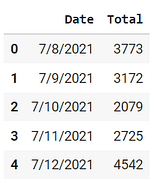(Image by Author)

``````# plot the data
data.plot()``````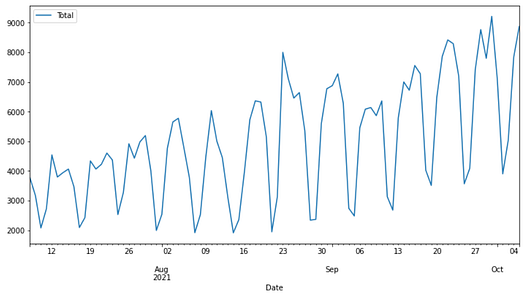This time series is of the number of daily downloads of the PyCaret library from pip.

## ⚙️ Initialize Setup

``````# with functional API
from pycaret.time_series import *
setup(data, fh = 7, fold = 3, session_id = 123)# with new object-oriented API
from pycaret.internal.pycaret_experiment import TimeSeriesExperiment
exp = TimeSeriesExperiment()
exp.setup(data, fh = 7, fold = 3, session_id = 123)``````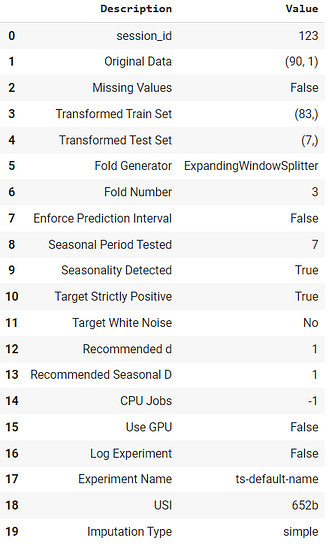(Image by Author) Output from the setup function

## 📐Statistical Testing

``check_stats()``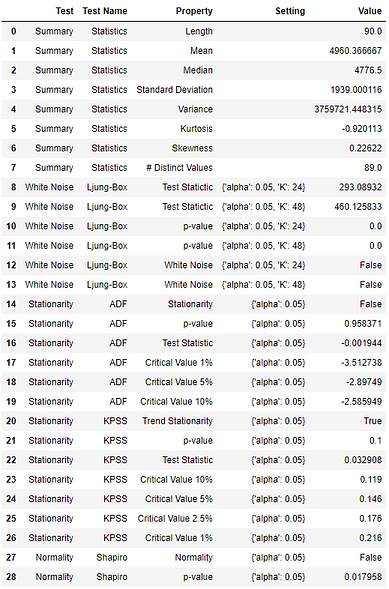(Image by Author) Output from the check_stats function

## 📈 Exploratory Data Analysis

``````# functional API
plot_model(plot = 'ts')# object-oriented API
exp.plot_model(plot = 'ts')``````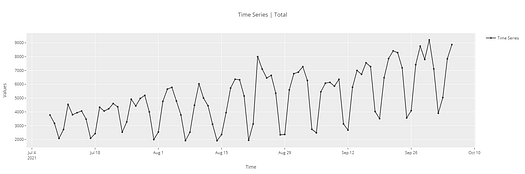(Image by Author)

``````# cross-validation plot
plot_model(plot = 'cv')``````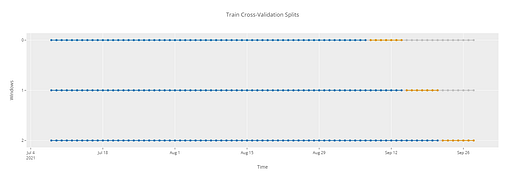(Image by Author)

``````# ACF plot
plot_model(plot = 'acf')``````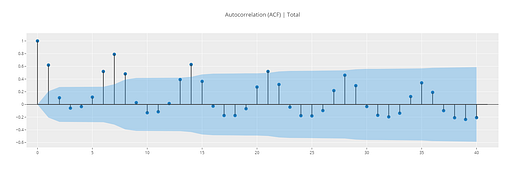``````# Diagnostics plot
plot_model(plot = 'diagnostics')``````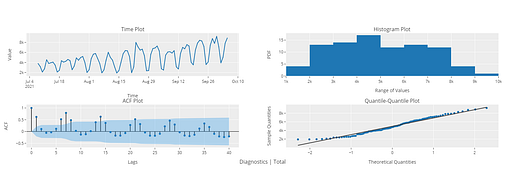``````# Decomposition plot
plot_model(plot = 'decomp_stl')``````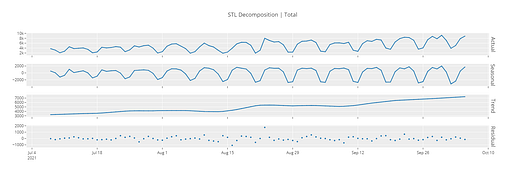## ✈️ Model Training and Selection

``````# functional API
best = compare_models()# object-oriented API
best = exp.compare_models()``````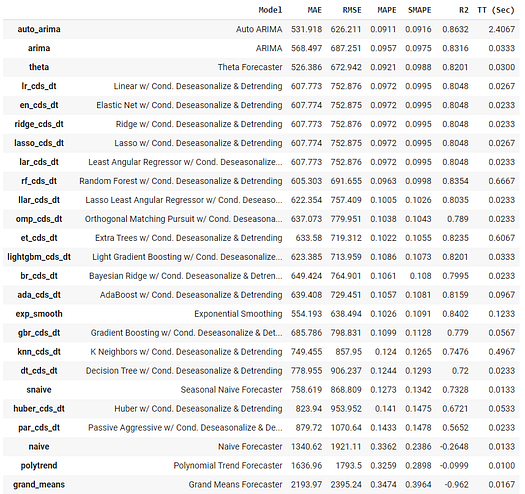(Image by Author) Output from the compare_models function

`create_model` in the time series module works just like it works in other modules.

``````# create fbprophet model
prophet = create_model('prophet')
print(prophet)``````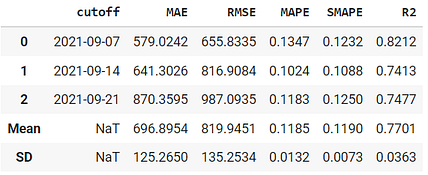(Image by Author) Output from the create_model function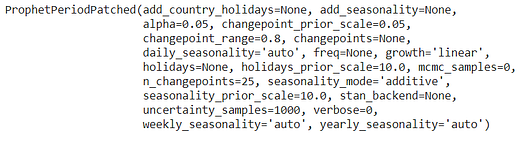(Image by Author) Output from the print function

`tune_model` isn’t much different either.

``````tuned_prophet = tune_model(prophet)
print(tuned_prophet)``````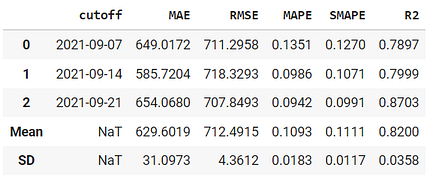(Image by Author) Output from the tune_model function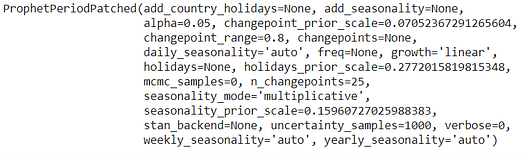(Image by Author) Output from the print function

``plot_model(best, plot = 'forecast')``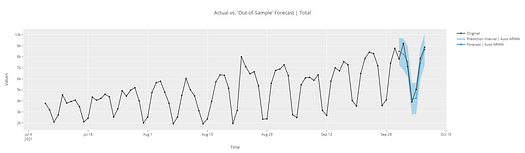(Image by Author)

``````# forecast in unknown future
plot_model(best, plot = 'forecast', data_kwargs = {'fh' : 30})``````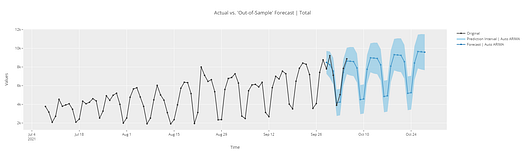(Image by Author)

``````# in-sample plot
plot_model(best, plot = 'insample')``````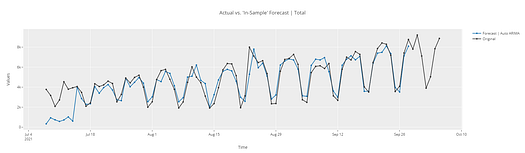``````# residuals plot
plot_model(best, plot = 'residuals')``````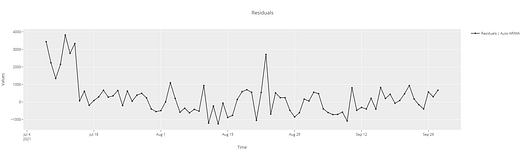``````# diagnostics plot
plot_model(best, plot = 'diagnostics')``````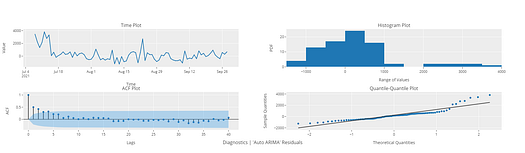## 🚀 Deployment

``````# finalize model
final_best = finalize_model(best)# generate predictions
predict_model(final_best, fh = 90)``````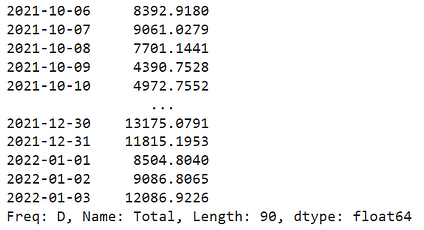(image by Author)

``````# save the model
save_model(final_best, 'my_best_model')``````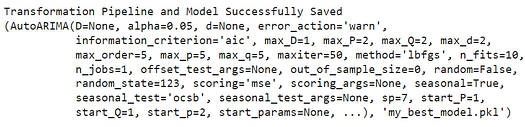(image by Author)

The module is still in beta. We are adding new functionalities every day and doing weekly pip releases. Please ensure to create a separate python environment to avoid dependency conflicts with the main pycaret. The final release of this module will be merged with the main pycaret in the next major release.

Developers:
Nikhil Gupta (lead), Antoni Baum Satya Pattnaik Miguel Trejo Marrufo Krishnan S G

There is no limit to what you can achieve using this lightweight workflow automation library in Python. If you find this useful, please do not forget to give us ⭐️ on our GitHub repository.

⭐ Tutorials New to PyCaret? Check out our official notebooks!
📋 Example Notebooks created by the community.
📙 Blog Tutorials and articles by contributors.
📚 Documentation The detailed API docs of PyCaret
📺 Video Tutorials Our video tutorial from various events.
📢 Discussions Have questions? Engage with community and contributors.
🛠️ Changelog Changes and version history.
🌳 Roadmap PyCaret’s software and community development plan.

Bio: Moez Ali writes about PyCaret and its use-cases in the real world, If you would like to be notified automatically, you can follow Moez on Medium, LinkedIn, and Twitter.

Original. Reposted with permission.

Related: